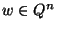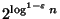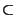Next: MAXIMUM KNAPSACK Up: Mathematical Programming Previous: MINIMUM UNSATISFYING LINEAR SUBSYSTEM   Index

### MAXIMUM HYPERPLANE CONSISTENCY

• INSTANCE: Finite sets P and N of integer n-vectors. P consists of positive examples and N of negative examples.

• SOLUTION: A hyperplane specified by a normal vectorand a bias.

• MEASURE: The number of examples that are consistent with respect to the hyperplane, i.e.,.

• Good News: Approximable within 2 .

• Comment: Variation in which only one type of misclassification, either positive or negative, is allowed is not approximable withinfor some. The complementary minimization problem, where the number of misclassifications is to be minimized, is not in APX unless P=NP, and is not approximable withinfor anyunless NPQP  and .